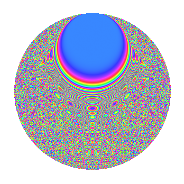# Properties

 Label 3024.2.efLevel 3024 Weight 2 Character orbit ef Rep. character $$\chi_{3024}(1027,\cdot)$$ Character field $$\Q(\zeta_{12})$$ Dimension 1024 Sturm bound 1152

# Related objects

## Defining parameters

 Level: $$N$$ = $$3024 = 2^{4} \cdot 3^{3} \cdot 7$$ Weight: $$k$$ = $$2$$ Character orbit: $$[\chi]$$ = 3024.ef (of order $$12$$ and degree $$4$$) Character conductor: $$\operatorname{cond}(\chi)$$ = $$112$$ Character field: $$\Q(\zeta_{12})$$ Sturm bound: $$1152$$

## Dimensions

The following table gives the dimensions of various subspaces of $$M_{2}(3024, [\chi])$$.

Total New Old
Modular forms 2352 1024 1328
Cusp forms 2256 1024 1232
Eisenstein series 96 0 96

## Trace form

 $$1024q + O(q^{10})$$ $$1024q - 32q^{16} - 24q^{22} + 12q^{28} - 60q^{40} + 36q^{52} + 48q^{58} - 48q^{64} - 64q^{67} + 192q^{70} + 60q^{82} - 32q^{88} - 24q^{91} - 36q^{94} + O(q^{100})$$

## Decomposition of $$S_{2}^{\mathrm{new}}(3024, [\chi])$$ into newform subspaces

The newforms in this space have not yet been added to the LMFDB.

## Decomposition of $$S_{2}^{\mathrm{old}}(3024, [\chi])$$ into lower level spaces

$$S_{2}^{\mathrm{old}}(3024, [\chi]) \cong$$ $$S_{2}^{\mathrm{new}}(112, [\chi])$$$$^{\oplus 4}$$$$\oplus$$$$S_{2}^{\mathrm{new}}(336, [\chi])$$$$^{\oplus 3}$$$$\oplus$$$$S_{2}^{\mathrm{new}}(1008, [\chi])$$$$^{\oplus 2}$$

## Hecke Characteristic Polynomials

There are no characteristic polynomials of Hecke operators in the database